[Mathematics] [Other] [Home]

Sphere Packing

Two possible ways to pack circles together on a plane are shown; hexagonal packing, which is the most efficient, and the less efficient method of putting the circles in a square array.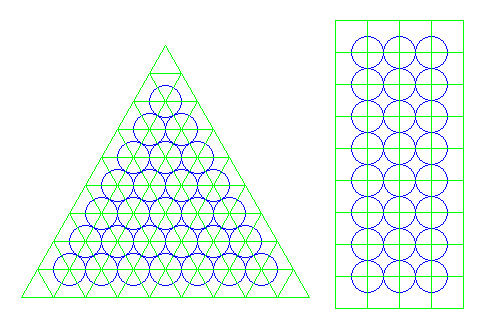Four spheres can touch each other, leaving little space between them, if they are arranged like the corners of a tetrahedron. However, unlike triangles, which tile the plane, tetrahedrons cannot fill space.

But there is a packing which fills space that involves alternating tetrahedrons and octahedrons.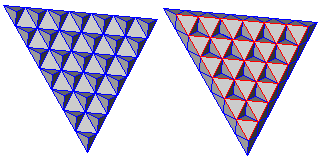First, on a plane divided in a tesselation of triangles, we place a tetrahedron on every second triangle, in a fashion similar to the way a checkerboard is colored.

Then the other triangles have a space above each of them into which an octahedron can fit neatly.

And once these spaces are filled, there are again spaces left which are perfect for another layer of tetrahedra, forming a flat slab.

If this is continued by placing tetrahedra on top of the octahedra to start the new layer, the result will be as symmetrical as possible, and the vertices of the polyhedra will be distributed in the same manner as spheres in a face-centered cubic packing.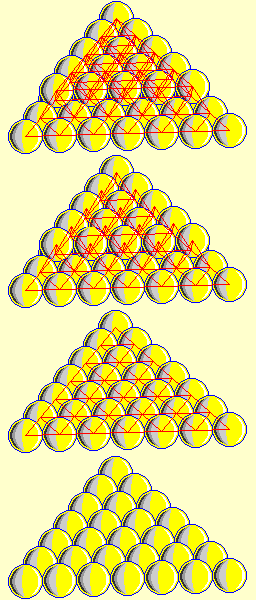This is illustrated by the diagram at left. Building from the bottom up, we see a triangular array of spheres sitting on the plane in hexagonal close packing.

Then, connecting the centers of the spheres, we see the tesellation of the plane with triangles.

Adding a second layer of spheres leads to their centers being joined to those of the layer below in lines which outline a set of tetrahedra, built on every second triangle.

Thus, if we join the apex of each tetrahedron to those of its neighbors, we outline octahedra and downwards pointing tetrahdra, just as in the polyhedral packing above.

Because we have octahedra in the polyhedral packing, and because octahedra have the same symmetry as cubes, and because the positions of the octahedra are in a regular array, we can take this close packing of spheres, and rotate it so that we see that the packing, instead of only having symmetries like those of a tetrahedron, can also be considered as resembling a cube. This is why it is known as the face-centered cubic packing, and when so oriented, it is even possible to assign each spere an integer position in Cartesian coordinates.

To illustrate this packing from that point of view, the diagram below shows one central sphere, and the twelve others which touch it, as assigned to cells in a cubical array: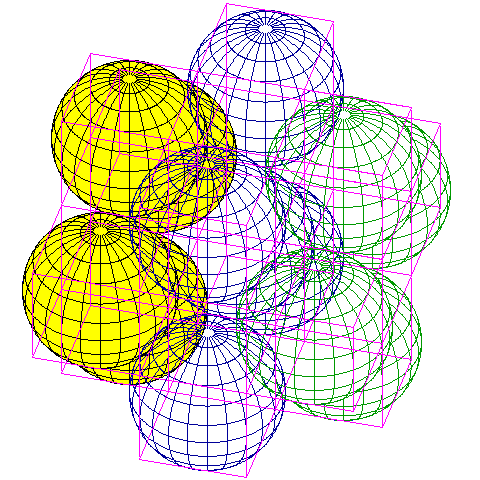It is obvious from this diagram that we are looking at spheres assigned to alternate cells in a three-dimensional array of cubes. But is this the same arrangement as we saw above? This may become clearer if we look at the spheres in a different order: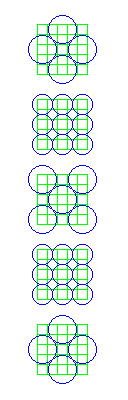Let us take this packing, and look at it in terms of the tesselation of cubes in which it is embedded, but let us shrink the cubes to half of their size, and view the packing in terms of multiple layers, stacked one above the other.

The reason for this is to allow us to conveniently think of what sphere packings might behave like in four or more dimensions. Naturally, the spheres have to be made into four-dimensional spheres as well, or the problem of packing them in four dimensions would be trivial, and the equivalent applies in higher dimensions.

By using cubes of half the size, we also happen to see the other points at which the spheres touch.

The convention used in the diagram on the left to illustrate the third dimension is also used in Fairy Chess (chess with non-standard rules, in general, not a particular variant form, specifically as used with chess problems) for diagrams for three-dimensional chess. The first two dimensions along which the spheres are positioned appear normally in each individual two-dimensional drawing; steps along the third dimension are indicated by moving from one vertically-stacked diagram to another.

When we look at how this packing might be extended into four dimensions, we see the following: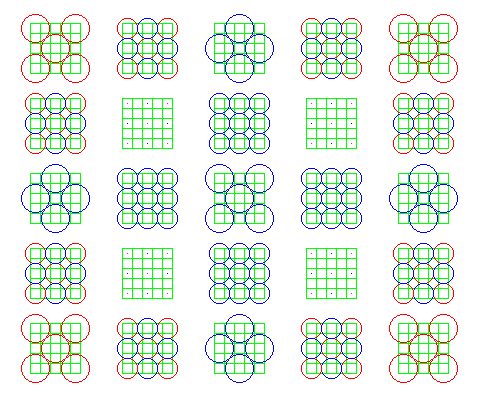in which the red circles represent spheres which do not touch the central sphere. Note that four of the plane slices do not contain any circles; but they do contain dots, which represent where spheres centered in boards shown diagonally opposite those boards in this diagram touch each other at a point.

Now, steps in the fourth dimension are indicated by moving horizontally, from one 3-D stack of 2-D diagrams to the next.

Thus, going to a higher dimension has created extra space in this packing. Reducing the scale of the diagram will allow a diagram showing the situation in six dimensions: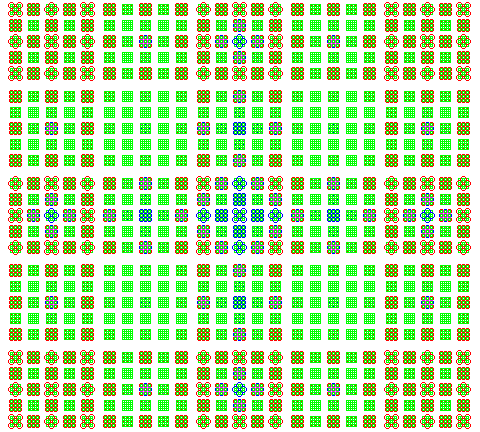Still more empty space has been created. Now we have a two-dimensional array of arrays of diagrams; just as position within each array indicated our position along the third and fourth dimensions, position of the array in the larger array of arrays indicates our position along the fifth and sixth dimensions. Since we will need to look at a sphere packing in eight dimensions, we will have to repeat this process one more time.

If we continue until we reach eight dimensions, there will be room to add new eight-dimensional spheres at points that are one square away from the central sphere in every one of the eight dimensions involved. This packing is known as the E8 lattice.

In that packing, 240 spheres touch the central sphere. 112 of them are spheres of the type we see now; 2 units from the central sphere in each of two dimensions, in either direction. The other 128 spheres are one unit away from the central sphere in every dimension. There are, of course, 256 possible positions that satisfy that description; given a Cartesian coordinate system, using only those positions where the number of plus signs for the directions is even will give a possible set of locations for those spheres. (Since one can reflect the lattice along one axis, odd parity will also do just as well.)

By showing both negative and positive displacements from the center in only the first four dimensions, and showing positive displacements only in the remaining four dimensions, it is possible to keep the diagram at a manageable size:Since positive displacements only are shown in four dimensions, only one-sixteenth of the possible 128 new spheres are shown in this diagram. The centers of these spheres are in the center of the diagram, in the cells with dark blue large circles. As with other spheres, the small circles reflect cells one space away from the centers in any dimensions except the first two (the circles themselves extend into the first two dimensions), and the dots reflect cells one space away from the centers in any two dimensions neither of which is one of the first two (a small circle would touch the center of the cell in the case where one of the dimensions is among the first two, and if both are, then a large circle would touch the center of the cell).

Incidentally, no attempt has been made to show where spheres of the new type, other than the sixteen shown, whose centers are outside the depicted area, touch cells in that area.

If we halve the size of the cubes again, and continue adding dimensions until we reach 24 dimensions, another opportunity to add many new spheres becomes available, leading to the Leech lattice. This lattice was discovered in 1965, and is closely related to the Golay code (previously discovered, in 1949).

The large diagram above, although drawn showing the individual spheres on a very small scale, does have a larger pattern which can be summarized further: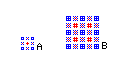In the diagram above, the area labelled A is a summary of the diagram above, and the one labelled B is a summary of what it would be with the negative displacements restored. Each pixel represents a four-dimensional array.

However, to continue on to 24 dimensions, one also has to halve the size of the basic cubes, doubling the number of layers, so it would be difficult to attempt to use a summary diagram of this sort to continue to higher dimensions in search of space for more spheres.

But if we formalize our process of making our small-scale summary diagrams just a bit, by using a specific color for each basic type of four-dimensional array: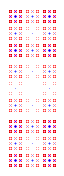then one might be able to press on to about fourteen dimensions or so, neglecting to double the scale, and using the summary diagram only as a rough guide. However, the diagram on the right shows an attempt of mine to proceed to eleven dimensions; I suspect, though, that I've overlooked some locations where I need to put spheres, or that I may do so if I continue on into another dimension, and it's hard to see what is going on using only a summary diagram of this type.

However, it isn't possible to find the Leech lattice quite as simply as that. There is a procedure almost as simple, since the Leech lattice is the laminated lattice of order 24, but one has to be willing to temporarily abandon having the spheres on integer coordinates during the intermediate steps of the process.

As we saw above, the E8 lattice includes two kinds of points. Of the 240 points which contact a sphere at the origin:

112 points are of the form

( +/- 2, +/- 2, 0, 0, 0, 0, 0, 0 )

where the signs are independent and the two nonzero displacements can be placed in any two dimensions, which correspond to the points in the face-centered-cubic packing in three dimensions and

128 points are of the form

( +/- 1, +/- 1, +/- 1, +/- 1, +/- 1, +/- 1, +/- 1, +/- 1 )

where the number of + signs must be even (or, again as noted, odd: as long as they are restricted in either fashion the lattice will be valid), and which are the new kind of points introduced for the E8 lattice.

Extending the E8 lattice the way the face-centered-cubic packing was extended simplistically to higher dimensions wouldn't yield the Leech lattice, because of the three kinds of points in that lattice, those that correspond to the new ones introduced with the E8 lattice are subject to a more complex restriction than would be yielded by trivially taking the E8 lattice to higher dimensions.

Now let us look at the three kinds of points found in the Leech lattice, by describing the coordinates of the points which are in contact with the central sphere. In this lattice, 196,560 points touch that sphere. And, as noted above, it is necessary to halve the size of the cubes, or double the size of the spheres, so our coordinates will be twice as large for points of corresponding type.

1,104 points are of the form:

( +/- 4, +/- 4, 0, 0, 0, 0, 0, 0,
0, 0, 0, 0, 0, 0, 0, 0,
0, 0, 0, 0, 0, 0, 0, 0 )

with no restriction on either of the signs, or the placement of the two nonzero coordinates among the dimensions. These points are obviously the ones corresponding to the points in the face-centered-cubic packing. And one can see that they are 2 * sqrt(2) units from the origin.

97,152 points are of the form:

( +/- 2, +/- 2, +/- 2, +/- 2, +/- 2, +/- 2, +/- 2, +/- 2,
0, 0, 0, 0, 0, 0, 0, 0,
0, 0, 0, 0, 0, 0, 0, 0 )

where the number of + signs must be even, so these points correspond to the new points added for the E8 lattice. These points are also 4 * sqrt(2) units from the origin. Since they have to be that far from each other as well (this is a packing of spheres whose radius is 2 * sqrt(2), after all), that means that there is a restriction on which dimensions can have nonzero coordinates. Any two vectors which do not have all their nonzero coordinates in the same places must have at least eight places in which one has a zero coordinate and the other has a nonzero coordinate. This is achieved by having the distributions of dimensions which are allowed correspond to the codewords in a binary Golay code including parity bit which have exactly eight ones in them. There are 759 such codewords. Thus, the Golay code makes its presence felt here, not only with the third kind of point.

98,304 points, finally, are of the form:

( +/- 3, +/- 1, +/- 1, +/- 1, +/- 1, +/- 1, +/- 1, +/- 1,
+/- 1, +/- 1, +/- 1, +/- 1, +/- 1, +/- 1, +/- 1, +/- 1,
+/- 1, +/- 1, +/- 1, +/- 1, +/- 1, +/- 1, +/- 1, +/- 1 )

For a given sign and position of the coordinate with the value 3, for the points of this form to be at least 4 * sqrt(2) units from each other, at least eight signs must be different from one point to the other. The sign of the 3 is independent of this, and it can be in any dimension. This is achieved by having the pattern of signs for the coefficients of absolute value 1 correspond to the pattern of 0 and 1 bits in a codeword in the binary Golay code without the parity bit. To keep points of the second and third kind far enough away from each other, there are other restrictions on which representation of the Golay code can be used in each case, so this description is not a full construction of the Leech lattice.

It can be seen from these restrictions that stopping at 11 dimensions in the summary diagram above was fortuitously correct, since it is at 12 dimensions that the influence of the Golay code first makes itself felt. At 12 dimensions, we can first add new points of the type added to make the E8 lattice.

To points of the form (111111110000), we can add points of the form (111100001111), by placing dark blue dots at the middle of the new diagram. However, if we do that, we also need to add points of the form (000011111111). Since these points now behave differently in the first four dimensions, the ones we've compressed to a single pixel, it is necessary to add a new series of pixel colors to the diagram. But as we continue adding more dimensions, we wind up having to add more different combinations within the first four dimensions, so this approach breaks down: at 14 dimensions, points of the form (11001100110011) are introduced, along with the linear combinations of that vector with the previous three.

[Mathematics] [Other] [Home]